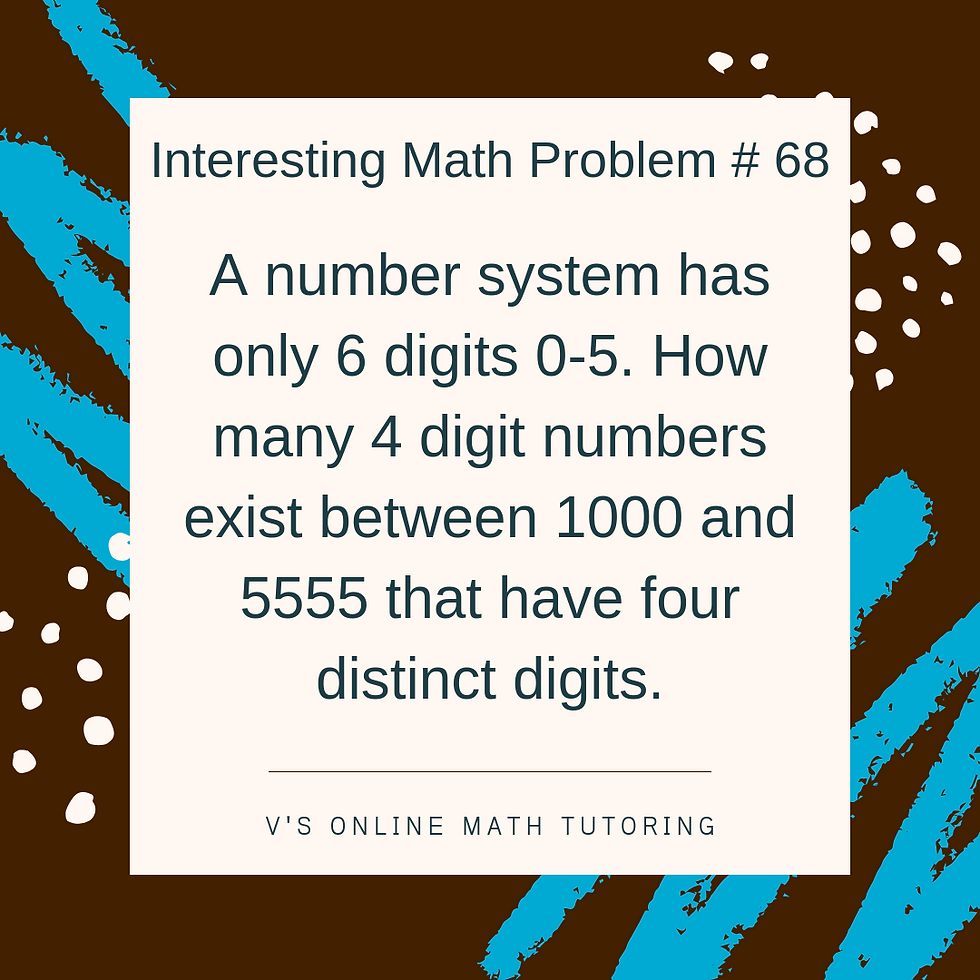top of pageSearch

# Math Competition Problems - Problem of the Week - 14

Updated: May 8, 2019

How many integers between 1000 and 9999 have four distinct digits? - AMC 8 2015We can try listing all the possibilities but that would take us almost forever! So we use the smarter approach.

Let's think of how many possible values each digit can take and then multiply them to get the total number of digits.

For eg. if you imagine a 2 digit number and the ten's digit has 9 choices and the unit's digit has 9 choices as well, then we can have a total of 81 numbers possible. That's because for each of those 9 choices in the ten's place, the unit's digit has 9 choices as well. So 9*9=81.

Now let's see how that works with our bigger problem as well.

We have digits from 0-9.

Our thousand's digit can take any digit from 1-9. It cannot be 0. Hence it has 9 choices.

The hundred's digit can take any digit from 0-9. That's 10 choices. But it cannot take the digit that is in the thousand's place. Hence it has 9 choices again.

The ten's digit can take any digit from 0-9. But it cannot take the digit in the thousand's or hundred's place. Hence it has 10-2=8 choices.

The unit's digit can take any digit from 0-9 as well. But it cannot take the digit in the previous three places. Hence it has 10-3=7 choices.

So the total possible numbers between 1000 and 9999 that have four distinct digits are

9*9*8*7 = 4536.

If you'd like to visualise how that happen's with an easier problem, here it is.

A number system has only 4 digits 0, 1, 2 and 3. How many 4 digit numbers exist between 1000 and 3333 that have four distinct digitsAs you can see in the above picture, we have 3 choices for the thousands digit - 1,2 and 3. We cannot start a number with 0.

We have 3 choices for our hundred's digit as well. How is that? It can be 0,1,2or 3. But it cannot have the number taken by the thousand's digit. Hence 4-1=3 choices.

Given that we've fixed our thousand's and hundred's digit, now our ten's digit cannot take the value that was taken by the previous 2 digits. Hence our ten's digit has 2 choices.

Now that all the other digit's are taken by thousand's, hundred's and ten's we need to use the remaining digit towards the unit's digit.

So for thousand's digit we have 3 choices.

For hundred's we have 3 choices as well.

For ten's we have 2 choices.

And the unit's digit has to take the remaining number - so no choice here.

So the number of 4 digit numbers with 4 distinct digits are 3*3*2*1=18. Which is also the same answer that we got from the number tree.

Now can you try a problem on your own?

A number system has only 6 digits 0-5. How many 4 digit numbers exist between 1000 and 5555 that have four distinct digits.Did you like the problem? Would you like to have these problems and solutions delivered to your inbox as I publish them? Click here to subscribe